# Algebra II : Vertical and Horizontal Lines

## Example Questions

← Previous 1 3

### Example Question #1 : Vertical And Horizontal Lines

Which of the following is a horizontal line?

Possible Answers: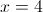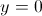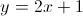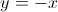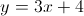Correct answer:Explanation:

A horizontal line has infinitely many values for, but only one possible value for. Thus, it is always of the form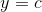, whereis a constant. Horizontal lines have a slope of. The only equation of this form is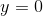### Example Question #2 : Vertical And Horizontal Lines

Which of the following equations represents a line that is perpendicular to?

Possible Answers: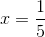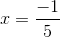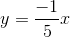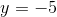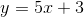Correct answer:Explanation:

The equationis a vertical line, so the perpendicular line must be horizontal. The only answer choice that is a horizontal line is.

### Example Question #3 : Vertical And Horizontal Lines

Which of the following is a vertical line?

Possible Answers: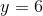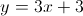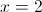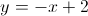Correct answer:Explanation:

A vertical line has infinitely many values ofbut only one value of. Thus, vertical lines are of the form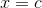, whereis a real number. The only equation of this form is### Example Question #4 : Vertical And Horizontal Lines

Which of the following answers describes the graph of this equation?(Select all answers that apply)

Possible Answers:

vertical line

Not enough information

horizontal line

Correct answer:

vertical line

Explanation:

The graph of x=5 is a vertical line. The equation x=5 represents all points with x- value equal to 5.

Try to plot a couple of points with an x-value of 5.

A few examples are (5, 0), (5, 2), (5,5).

Draw a line connecting the points and you obtain a vertical line intercepting the x-axis at (5,0).

### Example Question #5 : Vertical And Horizontal Lines

Which of the following is an equation of a vertical line?

Possible Answers: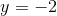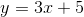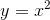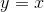Correct answer:Explanation:

Think about the meaning of a vertical line on the coordinate grid. Thevalue changes to any value, yet thevalue always stays the same. Thus, we are talking about an equation in which theis free, or is not effected, and theis constant. This is an equation of the form, whereis a constant.

### Example Question #6 : Vertical And Horizontal Lines

Which of the following is an equation of a horizontal line?

Possible Answers: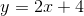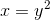Correct answer:Explanation:

Think about what it means to be a horizontal line. Thevalue changes to be any real number, but thevalue always remains constant. Thus, we are looking for an equation in which thevalue is constant and thevalue is not present. This would be any equation of the form, whereis a constant.

### Example Question #7 : Vertical And Horizontal Lines

I)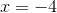II)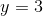III)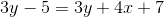IV)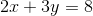Which of the presented equations are vertical lines?

Possible Answers:

I and II

II and IV

I and III

III and IV

I

Correct answer:

I and III

Explanation:

A vertical line is only dependent onand is completely independent of. Therefore, since I is, I is a vertical line. II is not a vertical line since it is only dependent upon; it is in fact a horizontal line.

For III, if we simplify the equation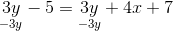it becomes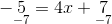and finally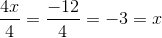Since III is only dependent upon, III is a vertical line.

IV is codependent upon bothand, so it is neither a vertical nor a horizontal line. Therefore, only I and III are vertical lines.

### Example Question #8 : Vertical And Horizontal Lines

What is the equation for the horizontal line containing the point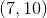?

Possible Answers: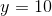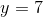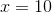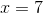Correct answer:Explanation:

The equation for a horizontal line is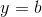where b is the y-coordinate of the point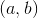on the line.

As such, the equation for the line containing the point (7, 10) is### Example Question #9 : Vertical And Horizontal Lines

What is the equation for the vertical line containing the point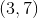?

Possible Answers:Correct answer:Explanation:

The equation for a vertical line is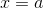whereis the-coordinate of the pointon the line.

As such, the equation for the line containing the pointis,.

### Example Question #10 : Vertical And Horizontal Lines

What is the slope of a vertical line?

Possible Answers:Undefined

Correct answer:

Undefined

Explanation:

The slope of a line is defined as the change in the y direction divided by the change in the x direction. A vertical line does not change in the y direction nor the x direction. This means that it's slope is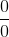. It is impossible to divide any number by zero, therefore the answer is undefined.

← Previous 1 3

### All Algebra II Resources